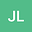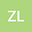Derivation and analysis of partial integro-differential inequality on shout options with its underlying asset subject to jump-diffusion model
••• Jun Liu,
• Zhian Liang
Jun Liu
Shanghai University of Finance and Economics

Corresponding Author:[email protected]

Author ProfileZhian Liang
Shanghai University of Finance and Economics
Author Profile## Abstract

Up to present, research on shout options remains only on the assumption that the underlying asset follows either Brownian motion or geometric Brownian motion. But it can not be evaluated accurately by PDE on geometric Brownian motion. To solve this problem this paper derives a new partial integro-differential inequality (PIDI) for shout options pricing on the assumption that the price of the underlying asset follows the jump-diffusion model and constructs the mathematical model by combining specific features and terminal conditions. On the basis of this model we obtain some results about shout options pricing. For this mathematical model this paper proposes a new competitive algorithm to choose two aspects. One is employing high-order difference for integral and partial derivative terms, the other is using Howard algorithm (also called policy iteration) for the complementarity problem. Numerical examples show that this algorithm yields an accurate technique and is more efficient than the traditional approaches in the case of geometric Brownian motion and jump-diffusion model, respectively.